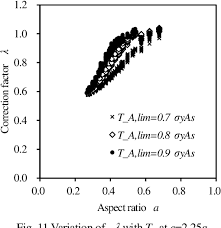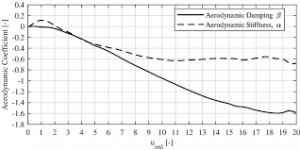## How to Calculate and Solve for Turbine Stiffness (Correction Factor) | Irrigation Water RequirementThe image above represents turbine stiffness.

To compute for turbine stiffness (correction factor), two essential parameters are needed and these parameters are Correction Factor for Radius of Curvature with Deflection (c) and Parallel Plate Stiffness for Tubing (σp).

The formula for calculating turbine stiffness:

σ = 0.149c[σp]

Where:

σ = Turbine Stiffness
c = Correction Factor for Radius of Curvature with Deflection
σp = Parallel Plate Stiffness for Tubing

Let’s solve an example;
Find the turbine stiffness when the correction factor for radius of curvature with deflection is 15 and the parallel plate stiffness for tubing is 3.

This implies that;

c = Correction Factor for Radius of Curvature with Deflection = 15
σp = Parallel Plate Stiffness for Tubing = 3

σ = 0.149c[σp]
σ = 0.149(15)
σ = 6.705

Therefore, the turbine stiffness is 6.705.

Calculating for the Correction Factor for Radius of Curvature with Deflection when the Turbine Stiffness and the Parallel Plate Stiffness for Tubing is Given.

c = σ / 0.149 x σp

Where;

c = Correction Factor for Radius of Curvature with Deflection
σ = Turbine Stiffness
σp = Parallel Plate Stiffness for Tubing

Let’s solve an example;
Find the correction factor for radius of curvature with deflection when the turbine stiffness is 32 and the parallel plate stiffness for tubing is 8.

This implies that;

σ = Turbine Stiffness = 32
σp = Parallel Plate Stiffness for Tubing = 8

c = σ / 0.149 x σp
c = 32 / 0.149 x 8
c = 32 / 1.192
c = 26.84

Therefore, the correction factor for radius of curvature with deflection is 26.84.

## How to Calculate and Solve for Turbine Stiffness | Irrigation Water RequirementThe image above represents turbine stiffness.

To compute for turbine stiffness, three essential parameters are needed and these parameters are Modulus of Elasticity for Tubing (E), Moment of Inertia of Tubing (I) and Mean Radius of Tubing (r).

The formula for calculating turbine stiffness:

σ = EI /

Where:

σ = Turbine Stiffness
E = Modulus of Elasticity for Tubing
I = Moment of Inertia of Tubing
r = Mean Radius of Tubing

Let’s solve an example;
Find the turbine stiffness when the modulus of elasticity for tubing is 12, the moment of inertia of tubing is 10 and the mean radius of tubing is 4.

This implies that;

E = Modulus of Elasticity for Tubing = 12
I = Moment of Inertia of Tubing = 10
r = Mean Radius of Tubing = 4

σ = EI /
σ = (12)(10) / (4)³
σ = 120 / 64
σ = 1.875

Therefore, the turbine stiffness is 1.875.

Calculating for the Modulus of Elasticity for Tubing when the Turbine Stiffness, the Moment of Inertia of Tubing and the Mean Radius of Tubing is Given.

E = σ x r³ / I

Where;

E = Modulus of Elasticity for Tubing
σ = Turbine Stiffness
I = Moment of Inertia of Tubing
r = Mean Radius of Tubing

Let’s solve an example;
Find the modulus of elasticity for tubing when the turbine stiffness is 20, the moment of inertia of tubing is 10 and the mean radius of tubing is 2.

This implies that;

σ = Turbine Stiffness = 20
I = Moment of Inertia of Tubing = 10
r = Mean Radius of Tubing = 2

E = σ x r³ / I
E = 20 x 2³ / 10
E = 20 x 8 / 10
E = 160 / 10
E = 16

Therefore, the modulus of elasticity for tubing is 16.